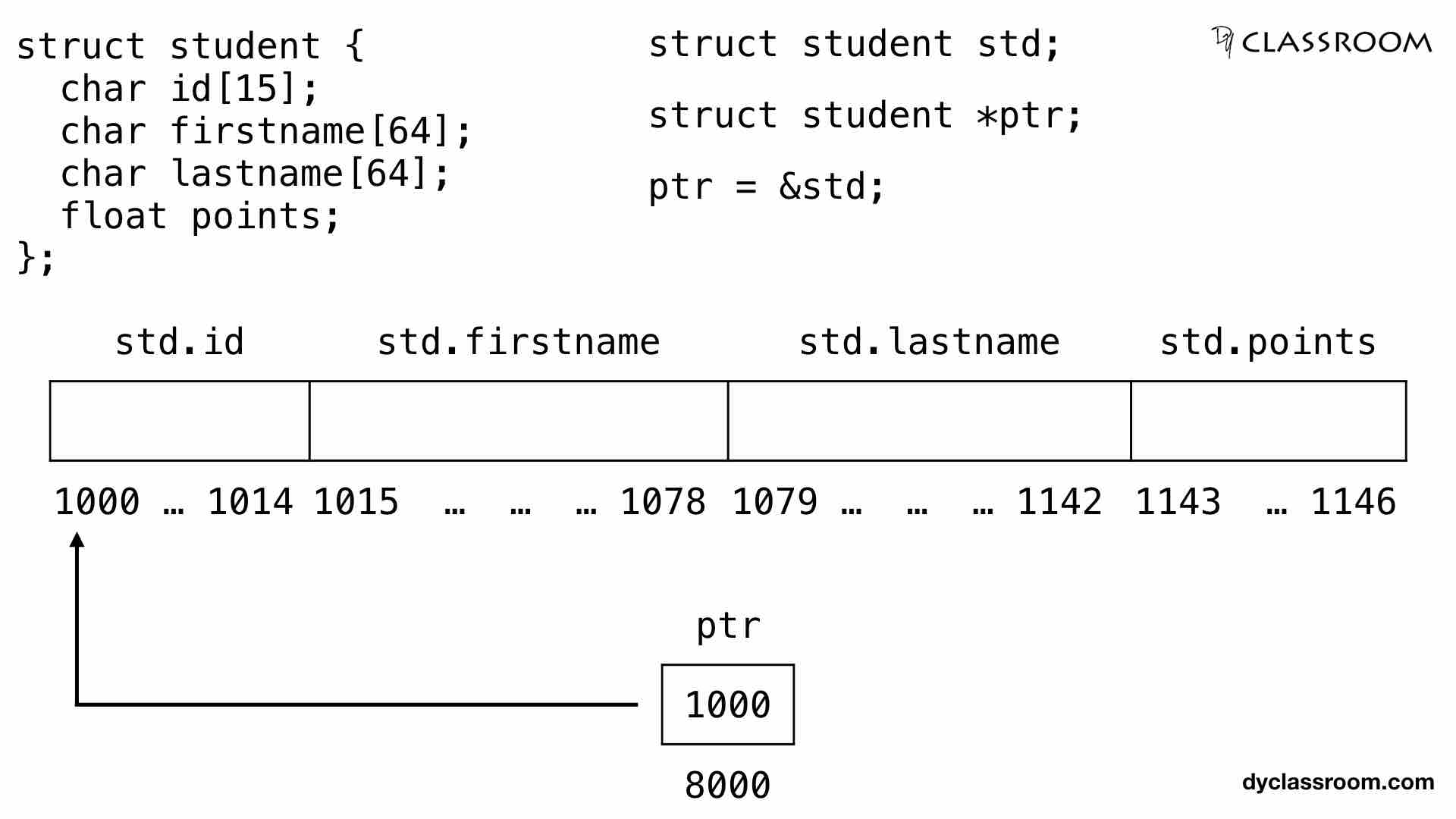# C - Pointers and Structures

C ProgrammingIn this tutorial we will learn to use pointers with structures in C programming language.

We have learned how to create and work with structures in the Structures tutorial. Feel free to check that out.

## Creating a structure

Lets start by creating a structure variable `student` as shown below.

``````// student structure
struct student {
char id;
char firstname;
char lastname;
float points;
};
``````

Now we will create a student structure variable `std`. For this we will write the following code.

``````// student structure variable
struct student std;
``````

## Access the members of a structure

We know that to access a member of a structure we use the `.` operator.

In the following example we are accessing the members of the student structure.

``````printf("ID: %s\n", std.id);
printf("First Name: %s\n", std.firstname);
printf("Last Name: %s\n", std.lastname);
printf("Points: %f\n", std.points);
``````

## Creating pointer for structure

Following is the syntax to create a pointer for a structure.

``````struct tagName *ptrName;
``````

So, to create a pointer for the student structure we will write the following code.

``````struct student *ptr;
``````

## Assigning structure variable to pointer

We use the following syntax to assign a structure variable address to a pointer.

``````ptrName = &structVarName;
``````

In the following example we are assigning the address of the structure variable `std` to the structure pointer variable `ptr`. So, ptr is pointing at std.

``````ptr = &std;
``````

## Accessing the members of a structure via pointer

We use the arrow operator also known as member selection operator `->` to access the members of a structure via pointer variable.

Following is the syntax for accessing members of a structure via pointer.

``````ptrName->member
``````

In the following example we are accessing the `firstname` member of the `student` structure via pointer variable `ptr`.

``````printf("First Name: %s", ptr->firstname);
``````

## Complete code

``````#include <stdio.h>

int main(void) {

// student structure
struct student {
char id;
char firstname;
char lastname;
float points;
};

// student structure variable
struct student std;

// student structure pointer variable
struct student *ptr = NULL;

// assign std to ptr
ptr = &std;

// get student detail from user
printf("Enter ID: ");
scanf("%s", ptr->id);
printf("Enter first name: ");
scanf("%s", ptr->firstname);
printf("Enter last name: ");
scanf("%s", ptr->lastname);
printf("Enter Points: ");
scanf("%f", &ptr->points);

// display result via std variable
printf("\nResult via std\n");
printf("ID: %s\n", std.id);
printf("First Name: %s\n", std.firstname);
printf("Last Name: %s\n", std.lastname);
printf("Points: %f\n", std.points);

// display result via ptr variable
printf("\nResult via ptr\n");
printf("ID: %s\n", ptr->id);
printf("First Name: %s\n", ptr->firstname);
printf("Last Name: %s\n", ptr->lastname);
printf("Points: %f\n", ptr->points);

return 0;
}
``````

Output:

``````Enter ID: s01
Enter first name: Yusuf
Enter last name: Shakeel
Enter Points: 8.44

Result via std
ID: s01
First Name: Yusuf
Last Name: Shakeel
Points: 8.440000

Result via ptr
ID: s01
First Name: Yusuf
Last Name: Shakeel
Points: 8.440000
``````

We can represent the student structure variable `std` in memory as follows.So, when we create the structure variable `std` it takes up 147 bytes of memory space.

MemberData TypeSizeMemory Location
idchar15 bytes1000 to 1014
firstnamechar64 bytes1015 to 1078
lastnamechar64 bytes1079 to 1142
pointsfloat4 bytes1143 to 1146

Next we have the pointer variable `ptr` that holds the memory address 1000 i.e., the memory address of the `std` structure variable. So, ptr is pointing at std.